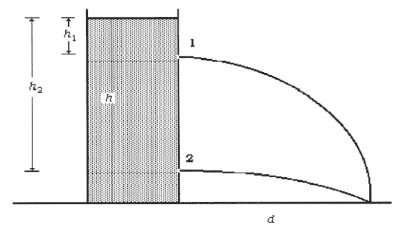# A cylinder full of water with two holes at different heights

Homework Statement:
To the sides of a container full of water there are two holes with a section negligible compared to the section of the base respectively at a distance of ##h_1=20 cm## and ##h_2=80cm## from the free surface of the water. Assuming that the tap pumps water in the container keeping constant the level of the liquid while water comes out from the holes and knowing that the two jet of water touch the ground in the same point (see picture), determine:
a) The height ##h## of the container;
b)the distance ##d## from the wall of the cylinder in which the holes are drilled and the point of the ground touched by the jets.
Relevant Equations:
-This is what I did:
I know that ##v_1=\sqrt{2gh_1}## and ##v_2=\sqrt{2gh_2}## thanks to Bernoulli's principle.
Using equations of parabolic motion I get
##h-h_1-\frac{1}{2}g(\frac{d}{v_1})^2=0## and ##h-h_1-\frac{1}{2}g(\frac{d}{v_2})^2=0##. This means I have two equations in two unknowns ##h## and ##d##.
That's it? I feel like I'm totally wrong but I don't see other ways.... (I checked dimension of both results)
the results are
$$d=\sqrt\frac{2(h_2-h_1)}{g(1/v_1^2-1/v_2^2)} = 0.8 m$$
and the height ##h=1.0 m##
Just let me know if you think I'm right :)

Doc Al
Mentor
Looks OK to me.

TSny
Homework Helper
Gold Member
Looks OK to me, too.

It's interesting to see how the expression ##d=\sqrt\frac{2(h_2-h_1)}{g(1/v_1^2-1/v_2^2)} ## simplifies if you make the substitutions ##v_1=\sqrt{2gh_1}## and ##v_2=\sqrt{2gh_2}##.

Likewise, ##h## has a simple expression in terms of ##h_1## and ##h_2##.

••ValeForce46, Delta2 and mfb
Looks OK to me, too.

It's interesting to see how the expression ##d=\sqrt\frac{2(h_2-h_1)}{g(1/v_1^2-1/v_2^2)} ## simplifies if you make the substitutions ##v_1=\sqrt{2gh_1}## and ##v_2=\sqrt{2gh_2}##.

Likewise, ##h## has a simple expression in terms of ##h_1## and ##h_2##.
You’re definitely right. At the end I get
##d=...=\sqrt{4h_1h_2}=0.8m##
Thank you!